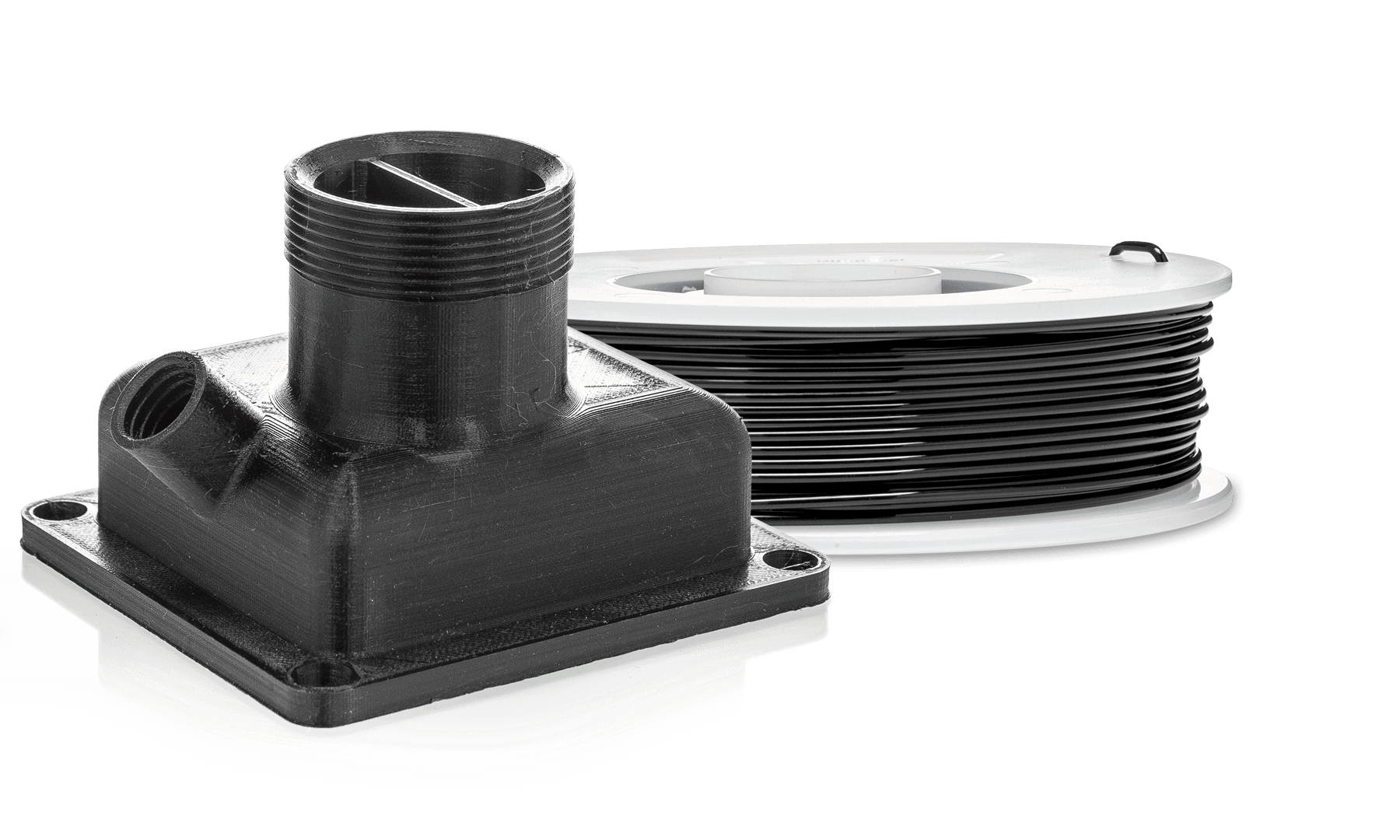``` \$( document ).ready(function() { \$(".imgbuy1").click(function(){ \$(".m1").show(); \$(".m2 , .m3 , .m4 , .m5 , .m6 , .m7 , .m8 , .m9 ,.m10 , .m11 , .m12 , .m13 ,.m14").hide(); // \$('.imgbuy1').addClass('myClass'); }); \$(".imgbuy2").click(function(){ \$(".m2").show(); \$(".m1 , .m3 , .m4 ,.m5 , .m6 , .m7 , .m8 , .m9 ,.m10 , .m11 , .m12 , .m13 ,.m14").hide(); }); \$(".imgbuy3").click(function(){ \$(".m3").show(); \$(".m2 , .m1 , .m4 ,.m5 , .m6 , .m7 , .m8 , .m9 ,.m10 , .m11 , .m12 , .m13 ,.m14").hide(); }); \$(".imgbuy4").click(function(){ \$(".m4").show(); \$(".m1 , .m3 , .m2 ,.m5 , .m6 , .m7 , .m8 , .m9 ,.m10 , .m11 , .m12 , .m13 ,.m14").hide(); }); \$(".imgbuy5").click(function(){ \$(".m5").show(); \$(".m1 , .m3 , .m4 ,.m2 , .m6 , .m7 , .m8 , .m9 ,.m10 , .m11 , .m12 , .m13 ,.m14").hide(); }); \$(".imgbuy6").click(function(){ \$(".m6").show(); \$(".m1 , .m3 , .m4 ,.m5 , .m2 , .m7 , .m8 , .m9 ,.m10 , .m11 , .m12 , .m13 ,.m14").hide(); }); \$(".imgbuy7").click(function(){ \$(".m7").show(); \$(".m1 , .m3 , .m4 ,.m5 , .m6 , .m2 , .m8 , .m9 ,.m10 , .m11 , .m12 , .m13 ,.m14").hide(); }); \$(".imgbuy8").click(function(){ \$(".m8").show(); \$(".m1 , .m3 , .m4 ,.m5 , .m6 , .m7 , .m2 , .m9 ,.m10 , .m11 , .m12 , .m13 ,.m14").hide(); }); \$(".imgbuy9").click(function(){ \$(".m9").show(); \$(".m1 , .m3 , .m4 ,.m5 , .m6 , .m7 , .m8 , .m2 ,.m10 , .m11 , .m12 , .m13 ,.m14").hide(); }); \$(".imgbuy10").click(function(){ \$(".m10").show(); \$(".m1 , .m3 , .m4 ,.m5 , .m6 , .m7 , .m8 , .m9 ,.m2 , .m11 , .m12 , .m13 ,.m14").hide(); }); \$(".imgbuy11").click(function(){ \$(".m11").show(); \$(".m1 , .m3 , .m4 ,.m5 , .m6 , .m7 , .m8 , .m9 ,.m10 , .m2 , .m12 , .m13 ,.m14").hide(); }); \$(".imgbuy12").click(function(){ \$(".m12").show(); \$(".m1 , .m3 , .m4 ,.m5 , .m6 , .m7 , .m8 , .m9 ,.m10 , .m11 , .m2 , .m13 ,.m14").hide(); }); \$(".imgbuy13").click(function(){ \$(".m13").show(); \$(".m1 , .m3 , .m4 ,.m5 , .m6 , .m7 , .m8 , .m9 ,.m10 , .m11 , .m12 , .m2 ,.m14").hide(); }); \$(".imgbuy14").click(function(){ \$(".m14").show(); \$(".m1 , .m3 , .m4 ,.m5 , .m6 , .m7 , .m8 , .m9 ,.m10 , .m11 , .m12 , .m13 ,.m2").hide(); }); }); ```